# state

UAV state vector

## Syntax

``stateVec = state(uavGuidanceModel)``

## Description

example

````stateVec = state(uavGuidanceModel)` returns a state vector for the specified UAV guidance model. The vector is always filled with zeros. Use this function to ensure you have the proper size for your state vector. Use the state vector as an input to the `derivative` function or when simulating the UAV using `ode45`.```

## Examples

collapse all

This example shows how to use the `multirotor` guidance model to simulate the change in state of a UAV due to a command input.

Create the multirotor guidance model.

`model = multirotor;`

Create a state structure. Specify the location in world coordinates.

```s = state(model); s(1:3) = [3;2;1];```

Specify a control command, `u`, that specified the roll and thrust of the multirotor.

```u = control(model); u.Roll = pi/12; u.Thrust = 1;```

Create a default environment without wind.

`e = environment(model);`

Compute the time derivative of the state given the current state, control command, and environment.

`sdot = derivative(model,s,u,e);`

Simulate the UAV state using `ode45` integration. The `y` field outputs the multirotor UAV states as a 13-by-n matrix.

```simOut = ode45(@(~,x)derivative(model,x,u,e), [0 3], s); size(simOut.y)```
```ans = 1×2 13 3536 ```

Plot the change in roll angle based on the simulation output. The roll angle (the X Euler angle) is the 9th row of the `simOut.y` output.

`plot(simOut.y(9,:))`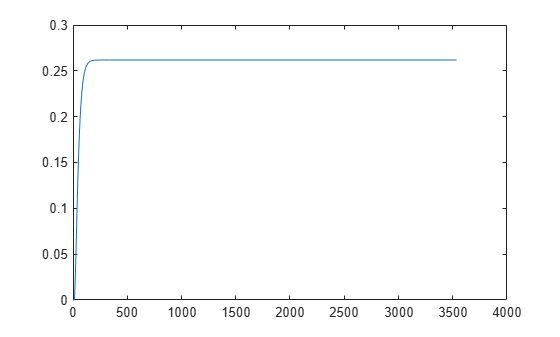Plot the change in the Y and Z positions. With the specified thrust and roll angle, the multirotor should fly over and lose some altitude. A positive value for Z is expected as positive Z is down.

```figure plot(simOut.y(2,:)); hold on plot(simOut.y(3,:)); legend('Y-position','Z-position') hold off```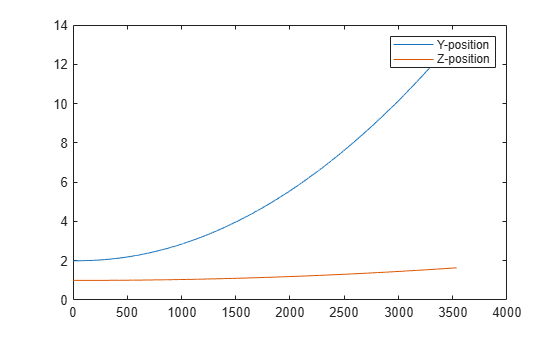You can also plot the multirotor trajectory using `plotTransforms`. Create the translation and rotation vectors from the simulated state. Downsample (every 300th element) and transpose the `simOut` elements, and convert the Euler angles to quaternions. Specify the mesh as the `multirotor.stl` file and the positive Z-direction as `"down"`. The displayed view shows the UAV translating in the Y-direction and losing altitude.

```translations = simOut.y(1:3,1:300:end)'; % xyz position rotations = eul2quat(simOut.y(7:9,1:300:end)'); % ZYX Euler plotTransforms(translations,rotations,... 'MeshFilePath','multirotor.stl','InertialZDirection',"down") view([90.00 -0.60])```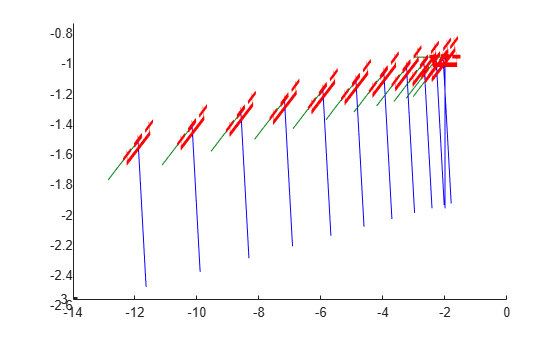This example shows how to use the `fixedwing` guidance model to simulate the change in state of a UAV due to a command input.

Create the fixed-wing guidance model.

`model = fixedwing;`

Set the air speed of the vehicle by modifying the structure from the `state` function.

```s = state(model); s(4) = 5; % 5 m/s```

Specify a control command, `u`, that maintains the air speed and gives a roll angle of `pi/12`.

```u = control(model); u.RollAngle = pi/12; u.AirSpeed = 5;```

Create a default environment without wind.

`e = environment(model);`

Compute the time derivative of the state given the current state, control command, and environment.

`sdot = derivative(model,s,u,e);`

Simulate the UAV state using `ode45` integration. The `y` field outputs the fixed-wing UAV states based on this simulation.

```simOut = ode45(@(~,x)derivative(model,x,u,e), [0 50], s); size(simOut.y)```
```ans = 1×2 8 904 ```

Plot the change in roll angle based on the simulation output. The roll angle is the 7th row of the `simOut.y` output.

`plot(simOut.y(7,:))`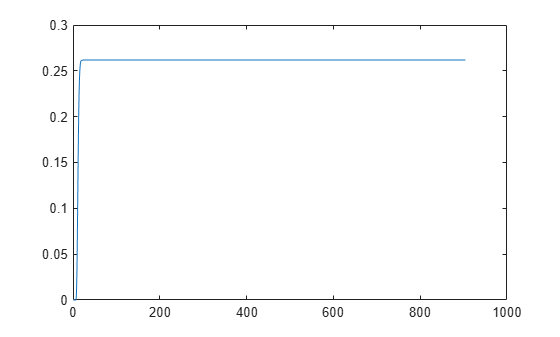You can also plot the fixed-wing trajectory using `plotTransforms`. Create the translation and rotation vectors from the simulated state. Downsample (every 30th element) and transpose the `simOut` elements, and convert the Euler angles to quaternions. Specify the mesh as the `fixedwing.stl` file and the positive Z-direction as `"down"`. The displayed view shows the UAV making a constant turn based on the constant roll angle.

```downsample = 1:30:size(simOut.y,2); translations = simOut.y(1:3,downsample)'; % xyz-position rotations = eul2quat([simOut.y(5,downsample)',simOut.y(6,downsample)',simOut.y(7,downsample)']); % ZYX Euler plotTransforms(translations,rotations,... 'MeshFilePath','fixedwing.stl','InertialZDirection',"down") hold on plot3(simOut.y(1,:),-simOut.y(2,:),simOut.y(3,:),'--b') % full path xlim([-10.0 10.0]) ylim([-20.0 5.0]) zlim([-0.5 4.00]) view([-45 90]) hold off```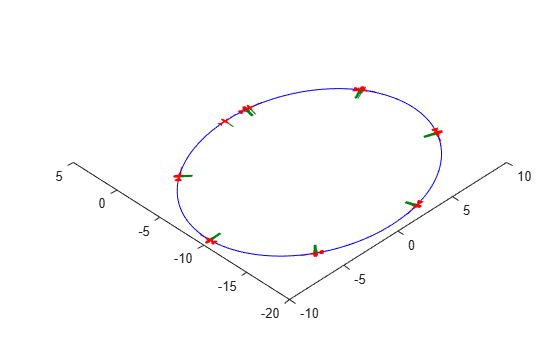## Input Arguments

collapse all

UAV guidance model, specified as a `fixedwing` or `multirotor` object.

## Output Arguments

collapse all

State vector, returned as a seven-element or thirteen-element vector. The vector is always filled with zeros. Use this function to ensure you have the proper size for your state vector.

For fixed-wing UAVs, the state is an eight-element vector:

• North - Position in north direction in meters.

• East - Position in east direction in meters.

• Height - Height above ground in meters.

• AirSpeed - Speed relative to wind in meters per second.

• HeadingAngle - Angle between ground velocity and north direction in radians.

• FlightPathAngle - Angle between ground velocity and north-east plane in radians.

• RollAngle - Angle of rotation along body x-axis in radians.

• RollAngleRate - Angular velocity of rotation along body x-axis in radians per second.

For multirotor UAVs, the state is a thirteen-element vector in this order:

• World Position - `[x y z]` in meters.

• World Velocity - `[vx vy vz]` in meters per second.

• Euler Angles (ZYX) - `[psi theta phi]` in radians.

• Body Angular Rates - `[p q r]` in radians per second.

• Thrust - `F` in Newtons.

## Extended Capabilities

### C/C++ Code GenerationGenerate C and C++ code using MATLAB® Coder™.

Introduced in R2018b

## SupportGet trial now